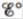# Calculate for the following half-reaction: AgI ( s ) + e − → Ag ( s ) + I − ( a q ) ( Hint: Reference the K sp value for AgI and the standard reduction potential for Ag + .)### Chemistry: An Atoms First Approach

2nd Edition
Steven S. Zumdahl + 1 other
Publisher: Cengage Learning
ISBN: 9781305079243

#### Solutions

Chapter
Section### Chemistry: An Atoms First Approach

2nd Edition
Steven S. Zumdahl + 1 other
Publisher: Cengage Learning
ISBN: 9781305079243
Chapter 17, Problem 91E
Textbook Problem
3 views

## Calculatefor the following half-reaction: AgI ( s ) + e − → Ag ( s ) + I − ( a q ) (Hint: Reference the Ksp value for AgI and the standard reduction potential for Ag+.)

Interpretation Introduction

Interpretation:

A half-cell reaction is given. The value of E° for the given cell reaction is to be calculated.

Concept introduction:

The relationship between reduction potential and standard reduction potential value and activities of species present in an electrochemical cell at a given temperature is given by the Nernst equation.

The value of Ecell is calculated using Nernst formula,

E=E°(RTnF)ln(Q)

At room temperature the above equation is specifies as,

E=E°(0.0591n)log(Q)

This relation is further used to determine the relation between ΔG° and K , ΔG° and E°cell .

Solubility product is applied only for those ionic compounds that are sparingly soluble. The product of solubility of ions is called solubility product and solubility is present in moles per liter.

To determine: The value of cell potential E° for the given cell reaction.

### Explanation of Solution

Given

The cell reaction is,

AgI(s)+eAg(s)+I(aq)

The value of standard reduction potential is,

Ag++eAgE°=0.80V

The value of solubility product constant is,

AgI(s)Ag+(aq)+I(aq)Ksp=8.3×1017

The reaction involves the transfer of 1 mole of electrons.

The standard cell potential at room temperature can be calculated by using the formula,

E°=0.0591nlogKsp

Where,

• Eo is the standard cell potential.
• n is the number of moles of electron transfer.
• Ksp is the solubility product constant.

Substitute the values of n and Ksp in the above equation,

E°=0.0591nlogKsp=0.05911log(8

### Still sussing out bartleby?

Check out a sample textbook solution.

See a sample solution

#### The Solution to Your Study Problems

Bartleby provides explanations to thousands of textbook problems written by our experts, many with advanced degrees!

Get Started

Find more solutions based on key concepts
Expalin the difference between cytosol and cytoplasm in both prokaryotes and eukaryotes.

Biology: The Unity and Diversity of Life (MindTap Course List)

How might nutritional genomics influence health care in the future?

Understanding Nutrition (MindTap Course List)

Which of the following describes the fat-soluble vitamins? a. few functions in the body b. easily absorbed and ...

Nutrition: Concepts and Controversies - Standalone book (MindTap Course List)

4.34 Define the term theoretical yield.

Chemistry for Engineering Students

Review. A 7.80-g bullet moving at 575 m/s strikes the hand of a superhero, causing the hand to move 5.50 cm in ...

Physics for Scientists and Engineers, Technology Update (No access codes included)

What is a coastal cell? Where does sand in a coastal cell come from? Where does it go?

Oceanography: An Invitation To Marine Science, Loose-leaf Versin